Matlab Toolbox - 5G/NR

PUCCH Format 0

NOTE :  It is required to have Matlab 5G Toolbox  to run this script. Matlab Version that I used for this page is 2019a v9.6, 5G Toolbox v1.1

 Ack Bit Variation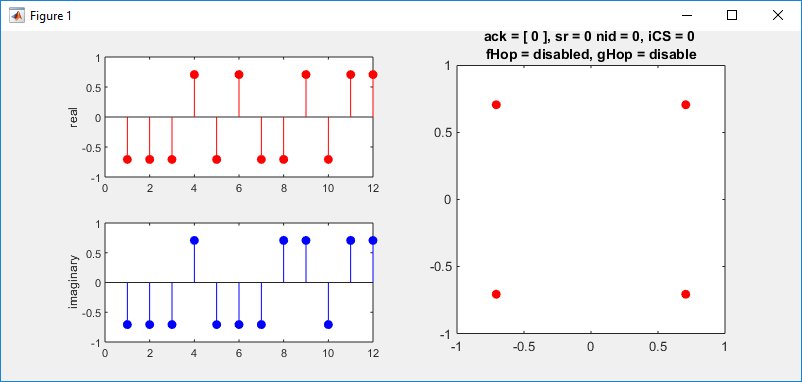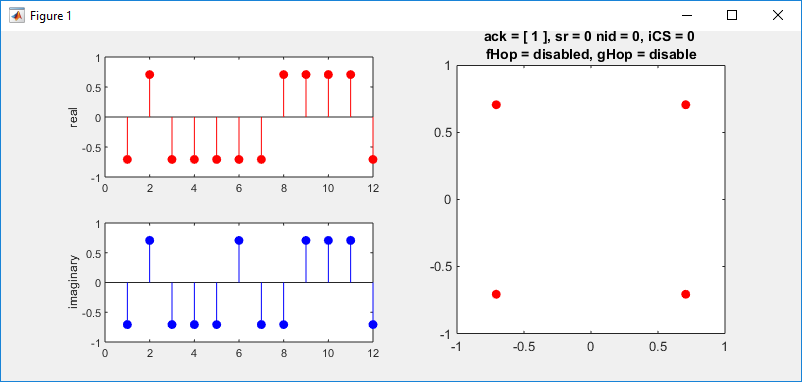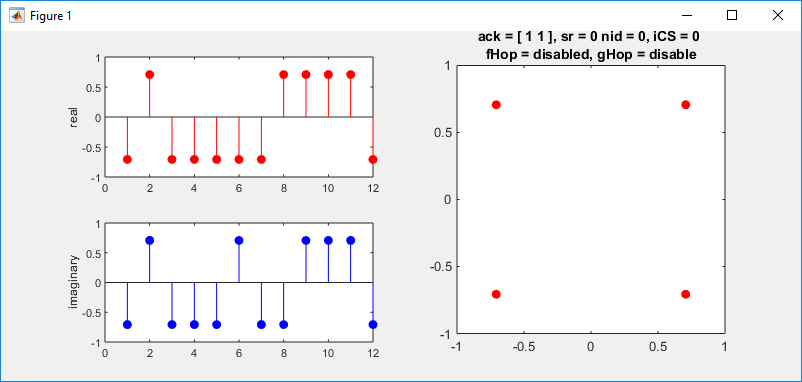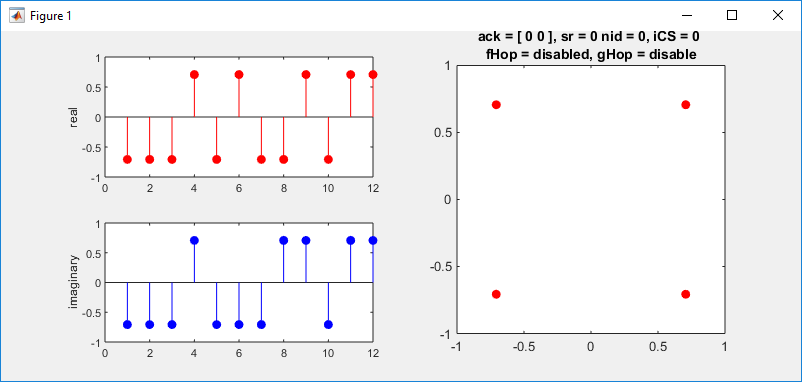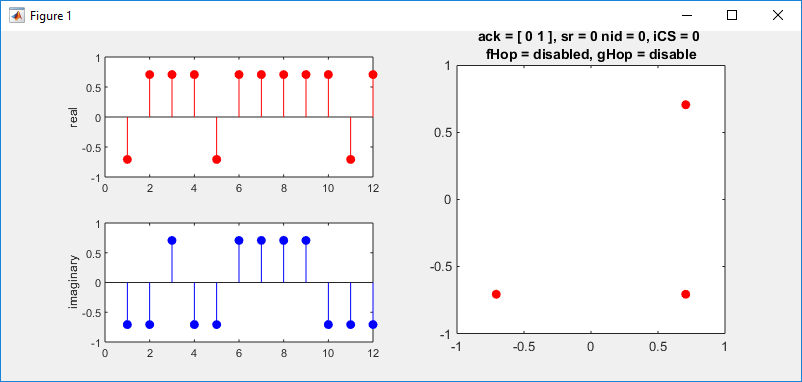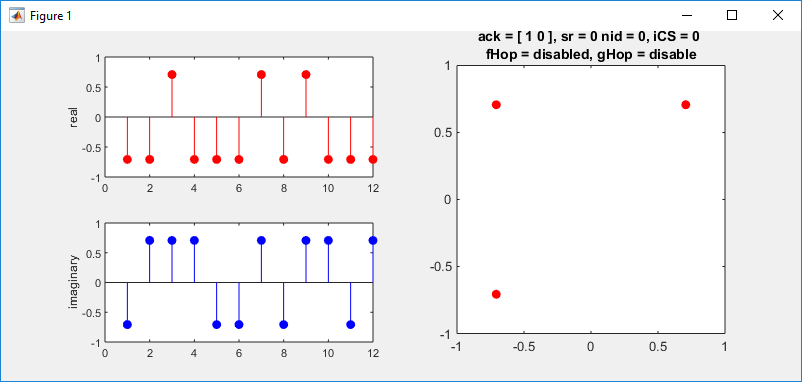SR Bit Variation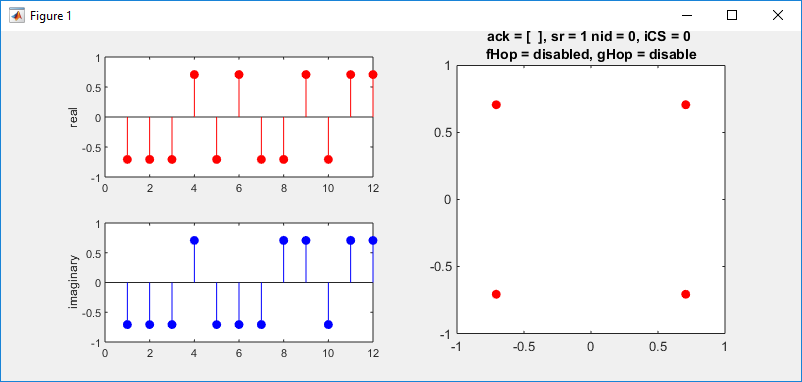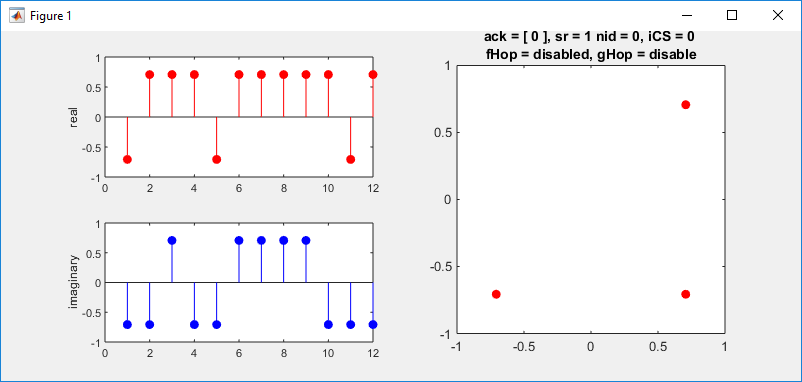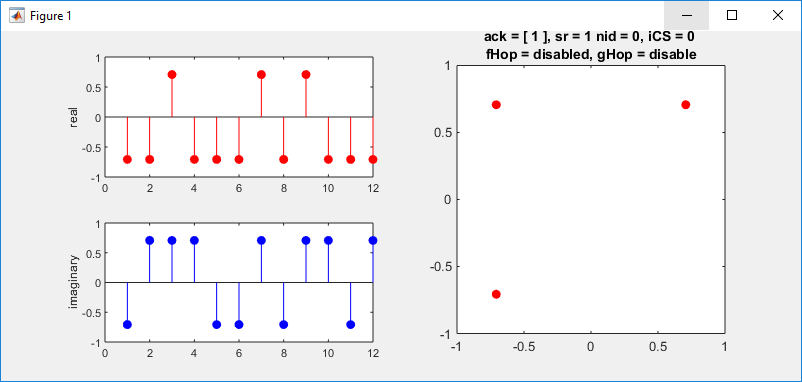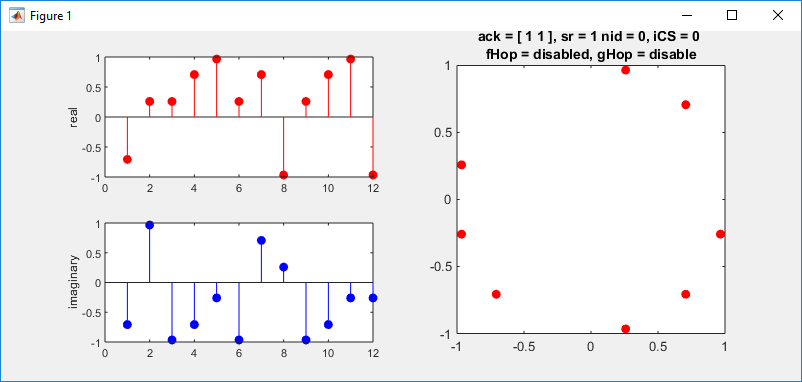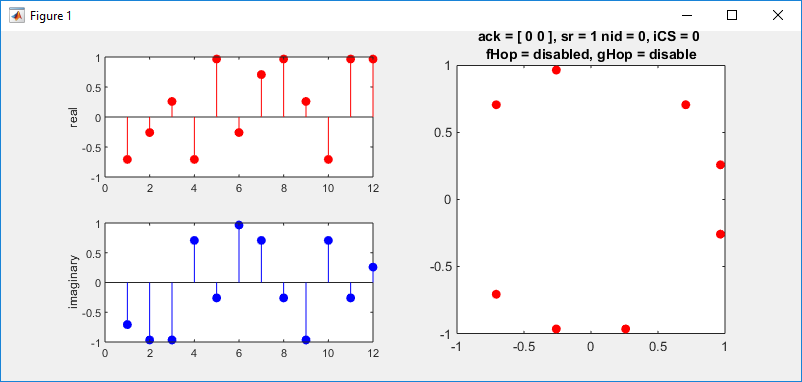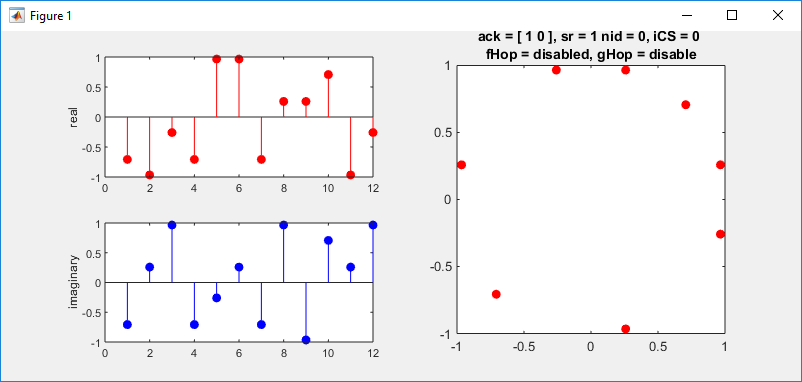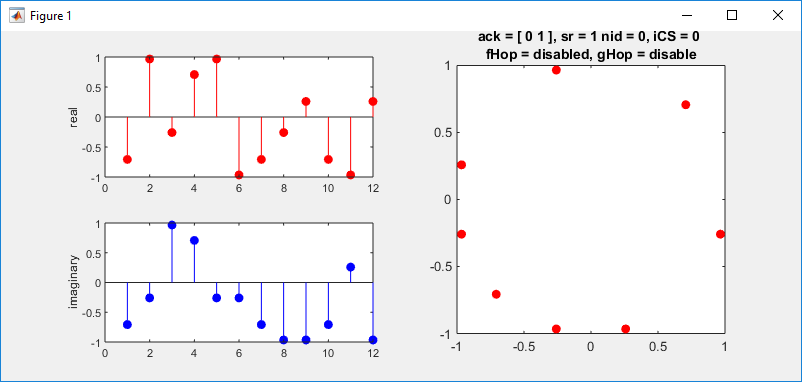InitialCS Variation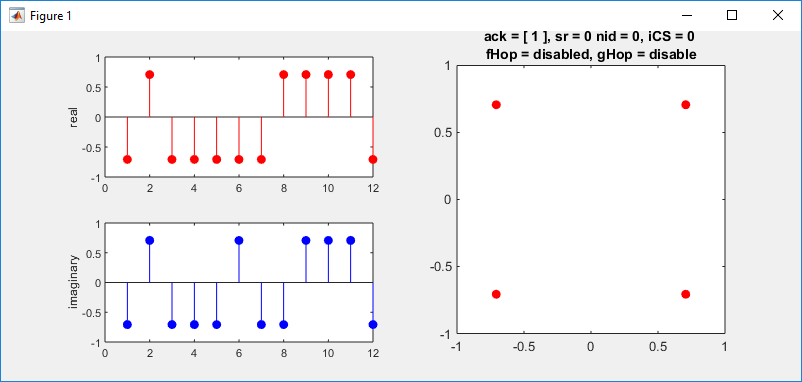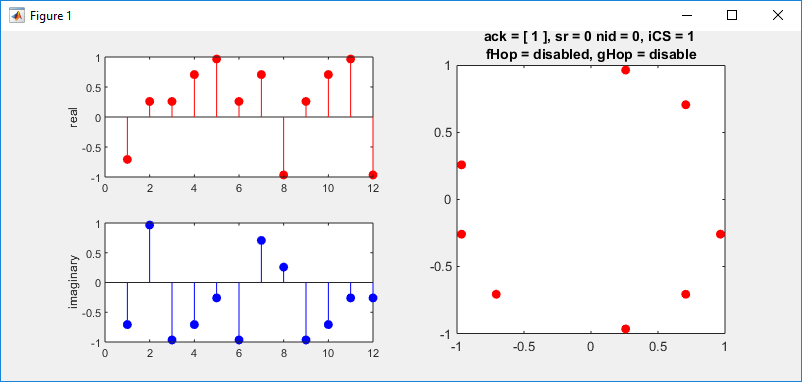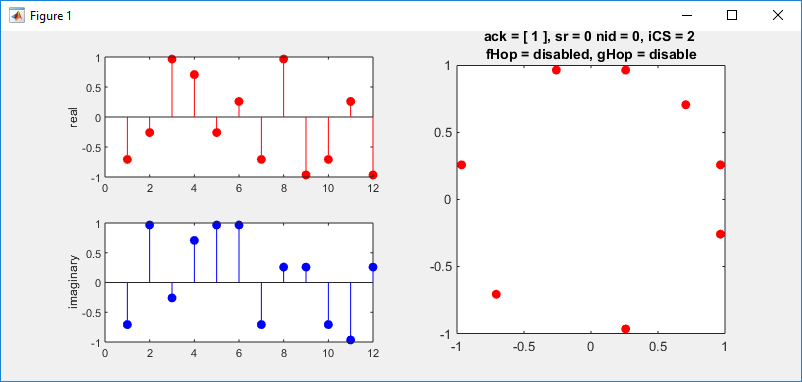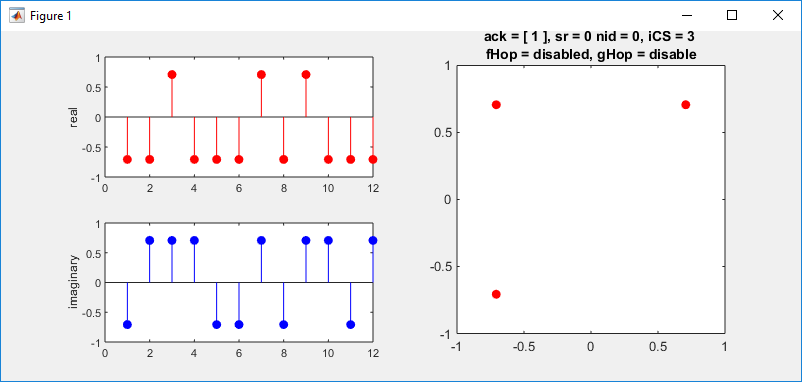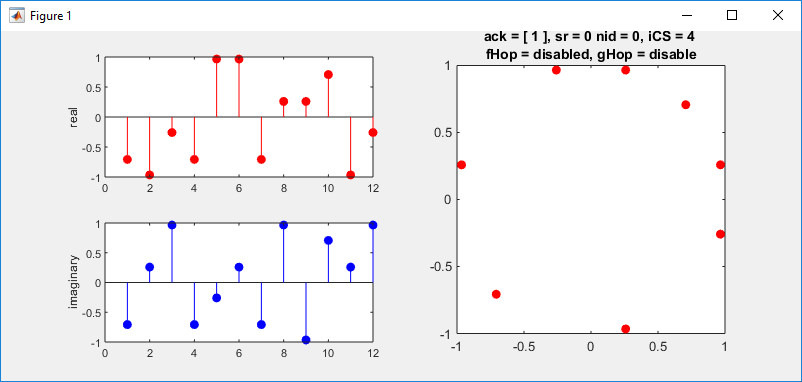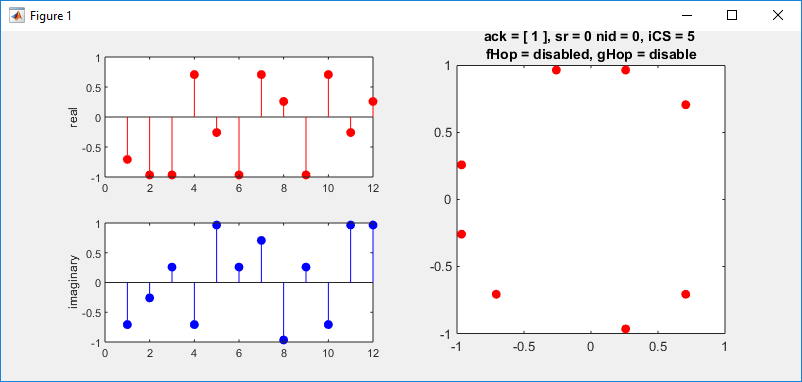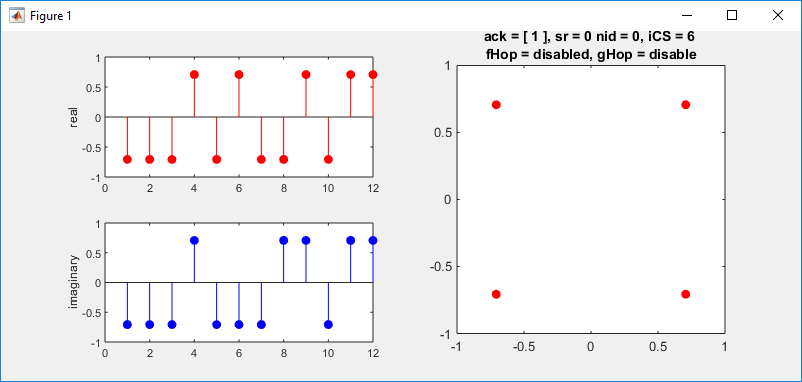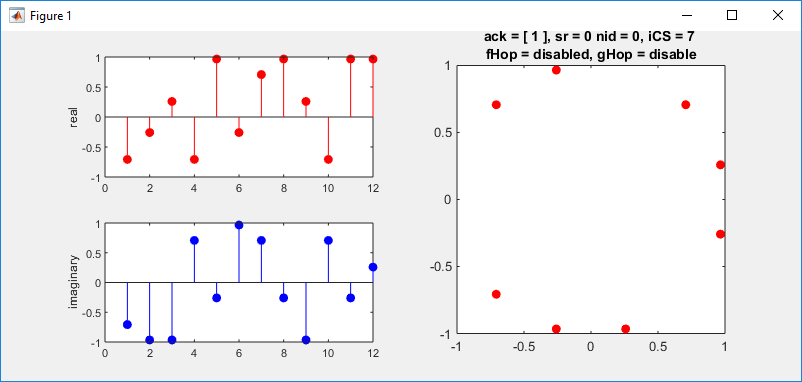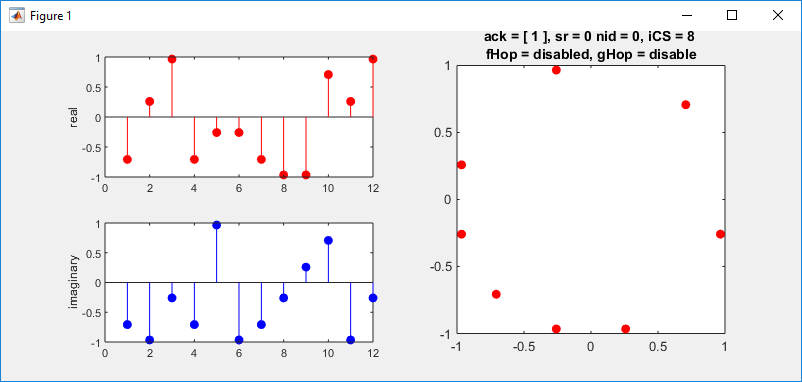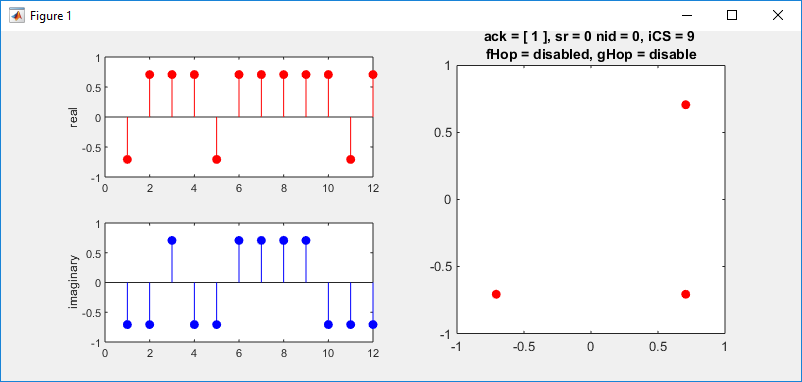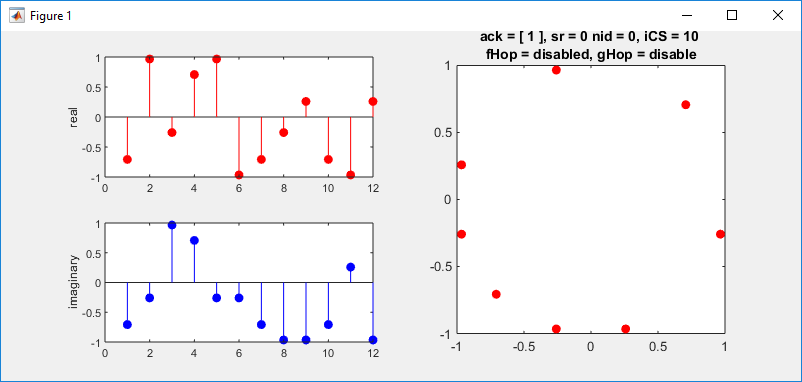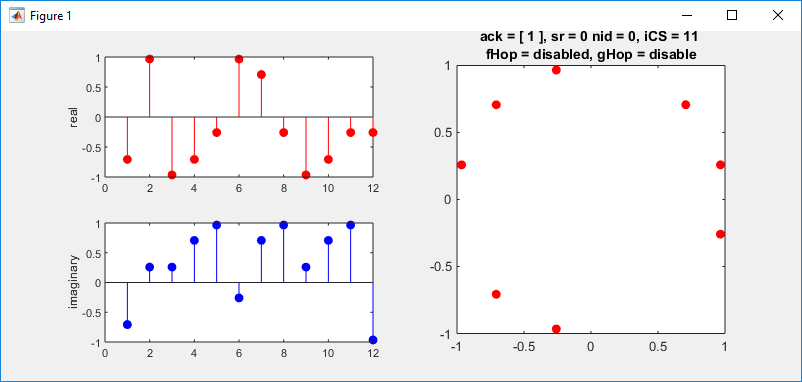Grouphopping Variation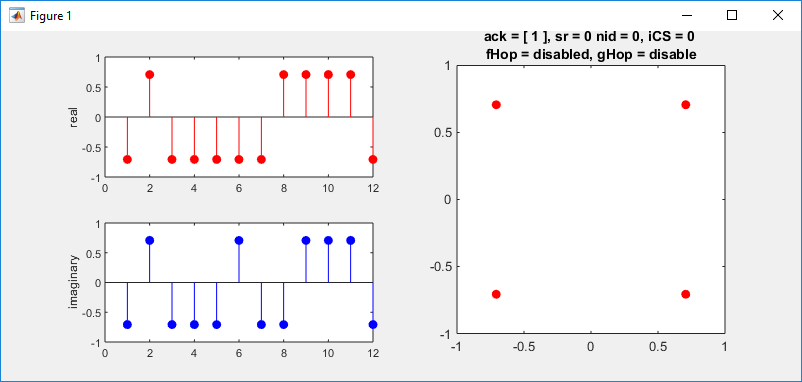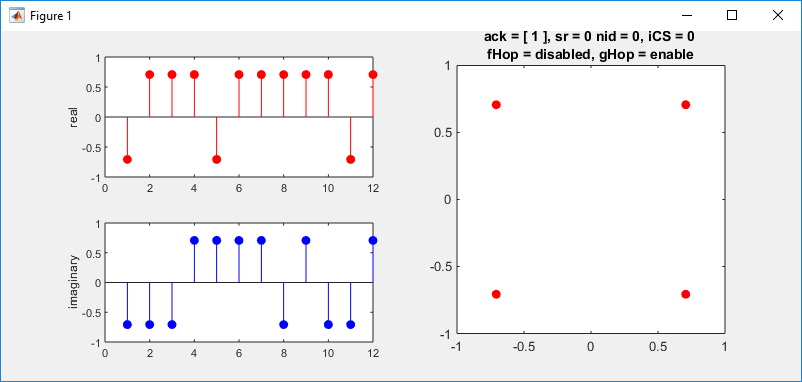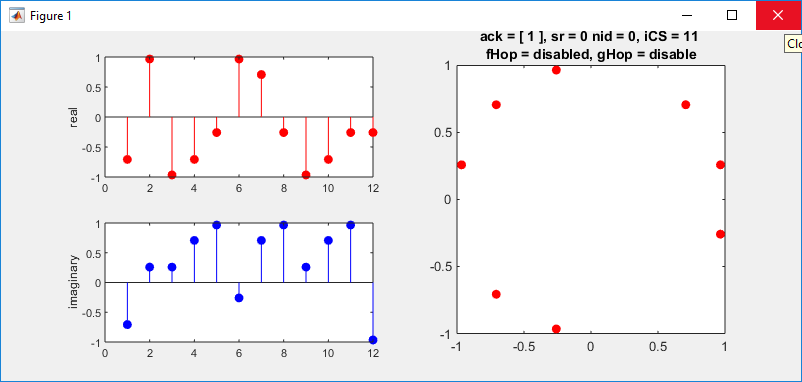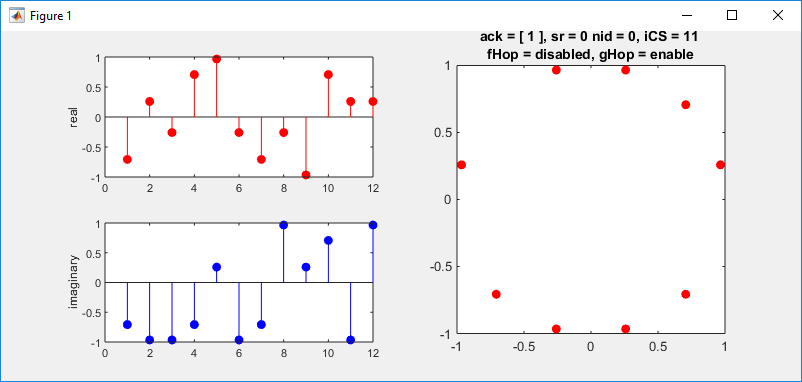Number of Symbol Variation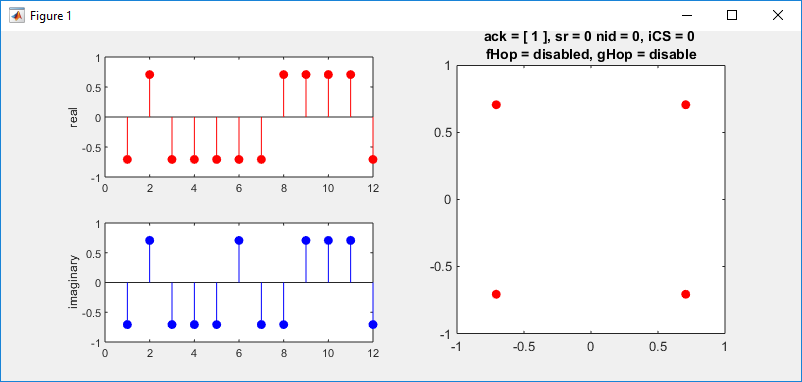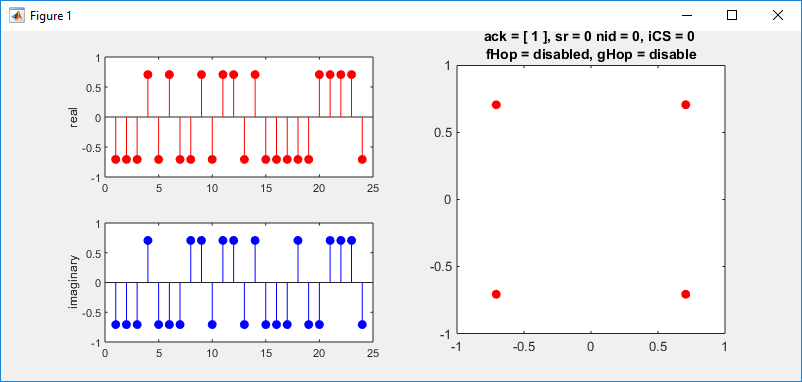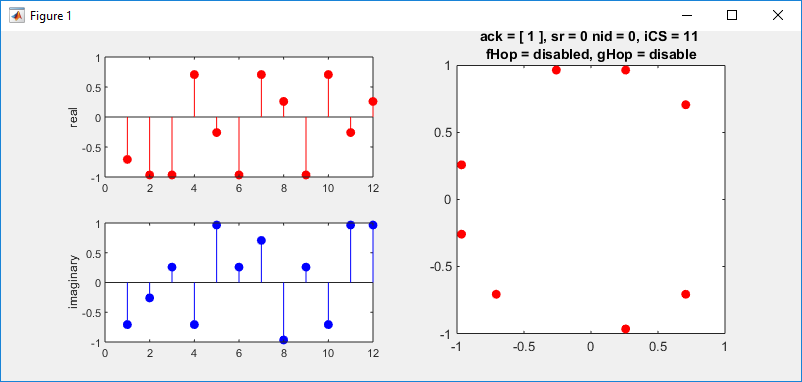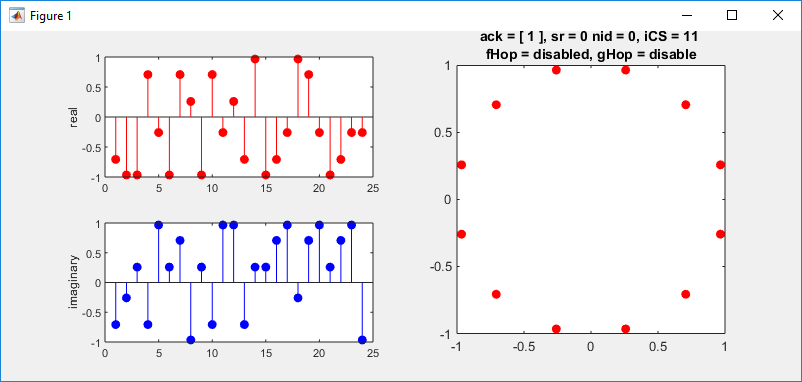Source Code

 5G_PUCCH_Format0.m ack = ; sr = 0; symAllocation = [13 1]; nslot = 0; nid = 12; initialCS = 0; cp = 'normal'; freqHopping = 'disabled'; groupHopping = 'disable'; sym = nrPUCCH0(ack,sr,symAllocation,cp,nslot,nid,groupHopping,initialCS,freqHopping)   h = figure(1);   subplot(2,2,1); stem(real(sym),'ro','MarkerFaceColor',[1 0 0]); ylabel('real');   subplot(2,2,3); stem(imag(sym),'bo','MarkerFaceColor',[0 0 1]); ylabel('imaginary');   subplot(2,2,[2 4]); plot(real(sym),imag(sym),'ro','MarkerFaceColor',[1 0 0]); axis([-1 1 -1 1]); titleText = sprintf('%d ',reshape(ack.',1,[])); titleText = sprintf('ack = [ %s], sr = %d',titleText, sr); titleText = sprintf('%s nid = %d, iCS = %d',titleText,nid,initialCS); titleText = sprintf('%s \nfHop = %s, gHop = %s',titleText,freqHopping,groupHopping); title(titleText); pbaspect([1 1 1]);   set(h,'Position',[400 200 800 350]); set(h, 'MenuBar', 'None')